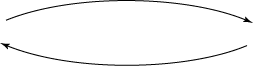# Mathematical methods for economic theory

Martin J. Osborne

## 7.5 Optimization: summary of conditions under which first-order conditions are necessary and sufficient

For all the results, f and gj for j = 1, ..., m are continuously differentiable functions of n variables and cj for j = 1, ..., m are constants.

### Unconstrained maximization problems

 x* solves maxx f(x)f'i(x*) = 0 for i = 1, ..., n if f is concave

### Equality-constrained maximization problems with one constraint

 if ∇g(x*) ≠ (0,...,0) x* solvesmaxx f(x) subject to g(x) = cthere exists λ such thatL'i(x*) = 0 for i = 1, ..., nand g(x*) = c if f is concave and λg is convex

where L(x) = f(x) − λ(g(x) − c).

### Inequality-constrained maximization problems

 if gj is concave for j = 1, ..., mor gj is convex for j = 1, ..., m and there exists x such that gj(x) < cj for j = 1, ..., mor gj is quasiconvex for j = 1, ..., m, ∇gj(x*) ≠ (0,...,0) for j = 1, ..., m, and there exists x such that gj(x) < cj for j = 1, ..., m x* solvesmaxx f(x) subject to gj(x) ≤ cj for j = 1, ..., mthere exists (λ1,...,λm) such thatL'i(x*) = 0 for i = 1, ..., nand λj ≥ 0, gj(x*) ≤ cj,and λj(gj(x*) − cj) = 0 for j = 1, ..., m if gj is quasiconvex for j = 1, ..., m and either f is concave or f is quasiconcave and ∇f(x*) ≠ (0,...,0)

where L(x) = f(x) − ∑m
j=1
λj(gj(x) − cj).